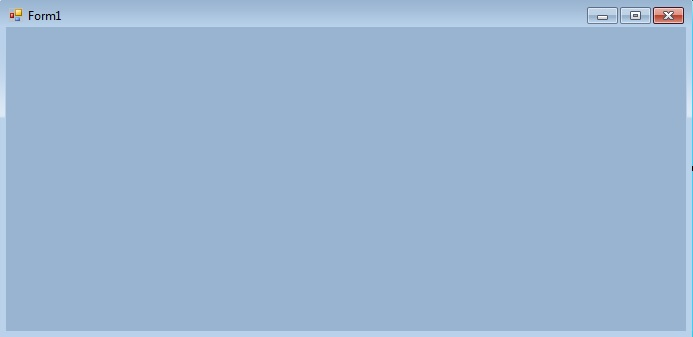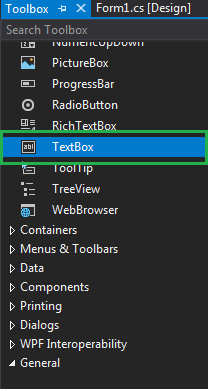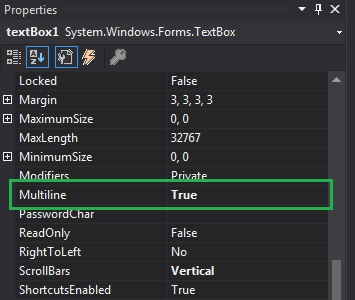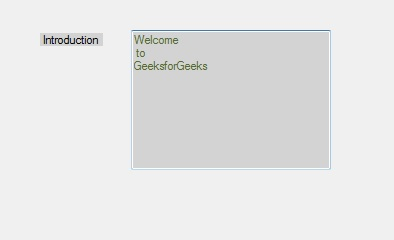Related Articles

# How to create Multiline TextBox in C#?

• Last Updated : 29 Nov, 2019

In Windows forms, TextBox plays an important role. With the help of TextBox, the user can enter data in the application, it can be of a single line or of multiple lines. In TextBox, you are allowed to create a multiline TextBox which stores multiple lines of the content using Multiline property of the TextBox. Set the value of this property to true from multiline TextBox, otherwise set false. The default value of this property is false. In Windows form, you can set this property in two different ways:

1. Design-Time: It is the simplest way to set the Multiline property of the TextBox as shown in the following steps:

• Step 1: Create a windows form.
Visual Studio -> File -> New -> Project -> WindowsFormApp• Step 2: Drag the TextBox control from the ToolBox and drop it on the windows form. You can place TextBox anywhere on the windows form according to your need.• Step 3: After drag and drop you will go to the properties of the TextBox control to set the Multiline property of the TextBox.Output:2. Run-Time: It is a little bit trickier than the above method. In this method, you can set the Multiline property of the TextBox programmatically with the help of given syntax:

`public override bool Multiline { get; set; }`

Here, the value of this property is of System.Boolean type. Following steps are used to set the Multiline property of the TextBox:

• Step 1 : Create a textbox using the TextBox() constructor provided by the TextBox class.
```// Creating textbox
TextBox Mytextbox = new TextBox();
```
• Step 2 : After creating TextBox, set the Multiline property of the TextBox provided by the TextBox class.
```// Set Multiline property
Mytextbox.Multiline = true;
```
• Step 3 : And last add this textbox control to from using Add() method.
```// Add this textbox to form
 `using` `System;``using` `System.Collections.Generic;``using` `System.ComponentModel;``using` `System.Data;``using` `System.Drawing;``using` `System.Linq;``using` `System.Text;``using` `System.Threading.Tasks;``using` `System.Windows.Forms;`` ` `namespace` `my {`` ` `public` `partial` `class` `Form1 : Form {`` ` `    ``public` `Form1()``    ``{``        ``InitializeComponent();``    ``}`` ` `    ``private` `void` `Form1_Load(``object` `sender, EventArgs e)``    ``{`` ` `        ``// Creating and setting the properties of Lable1``        ``Label Mylablel = ``new` `Label();``        ``Mylablel.Location = ``new` `Point(96, 54);``        ``Mylablel.Text = ``"Introduction"``;``        ``Mylablel.AutoSize = ``true``;``        ``Mylablel.BackColor = Color.LightGray;`` ` `        ``// Add this label to form``        ``this``.Controls.Add(Mylablel);`` ` `        ``// Creating and setting the properties of TextBox1``        ``TextBox Mytextbox = ``new` `TextBox();``        ``Mytextbox.Location = ``new` `Point(187, 51);``        ``Mytextbox.BackColor = Color.LightGray;``        ``Mytextbox.ForeColor = Color.DarkOliveGreen;``        ``Mytextbox.Height = 140;``        ``Mytextbox.Width = 200;``        ``Mytextbox.Name = ``"text_box1"``;``        ``Mytextbox.Multiline = ``true``;`` ` `        ``// Add this textbox to form``        ``this``.Controls.Add(Mytextbox);``    ``}``}``}`